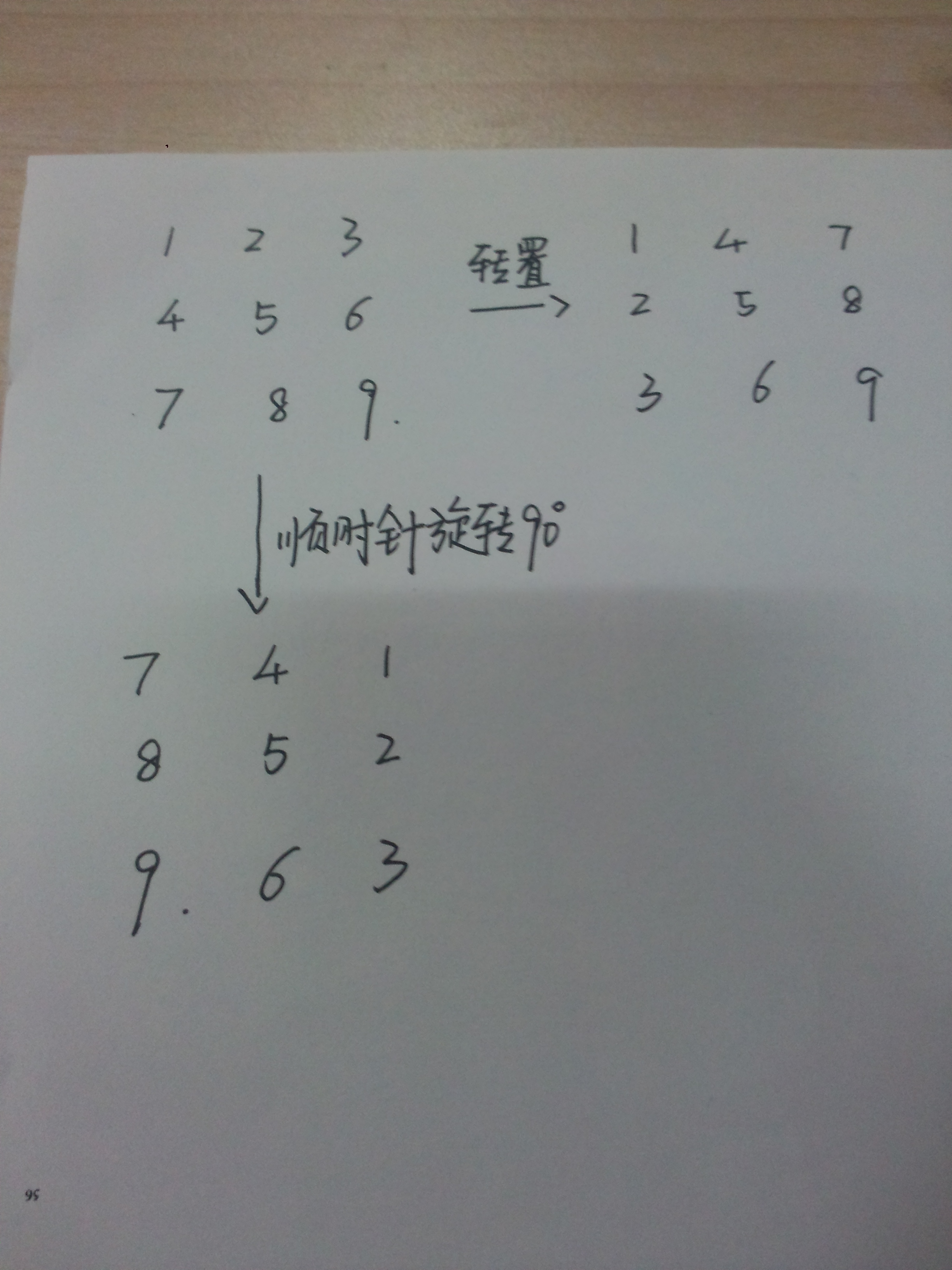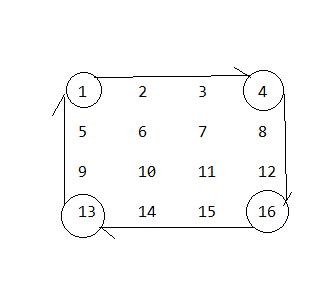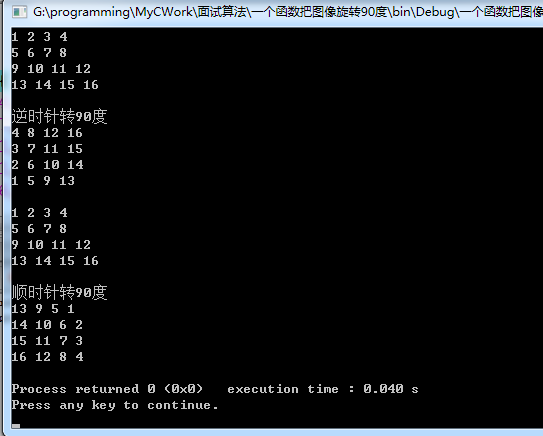• 2020-09-16 18:36:59

## 以下是c语言的矩阵旋转90度的代码

#include <iostream>
#include <stdio.h>
#include <math.h>
#include <algorithm>
#include <vector>
#include <deque>
#include <string>
#include <stack>
#include <queue>
#include <list>
#include <set>
#include <map>
#include <stdlib.h>
using namespace std;

#define buf_width (3)
#define buf_height (3)
char val[buf_width][buf_width] = {
{ 0,0,0 },
{ 0,0,0 },
{ 0,0,0 },
};
char Buffer[buf_width][buf_height] =
{
{ 1,2,3 },
{ 4,5,6 },
{ 7,8,9 },
};
void merg(char arry[])
{
int i, j, temp;
for (int i = 0; i < buf_width; i++) {
for (int j = 0; j < buf_width; j++) {
val[j][buf_width - 1 - i] = arry[i][j];
}
}
}
int main() {
void merg(char arry[]);
int i, j;

printf("原始的矩阵为：\n");
for (i = 0; i<buf_width; i++) {
for (j = 0; j<buf_width; j++) {
printf("%d ", Buffer[i][j]);
}
printf("\n");
}
printf("\n");
merg(Buffer);
printf("变换后的矩阵为：\n");
for (i = 0; i<buf_width; i++) {
for (j = 0; j<buf_width; j++) {
printf("%d ", val[i][j]);
}
printf("\n");
}
return 0;

}


更多相关内容
• ## 矩阵旋转90度

千次阅读 2018-01-14 13:07:45
//旋转90度   public static void rotate(int temp[][]){   int len=temp.length;   int b [][]=new int [len][len];   for(int i=0;i;i++){   for(int j=0;j;j++){   b...

一维数组转置（首尾交换）

[plain]  view plain
1. public class array {
2.     public static void main(String args[]){
3.         int data [] =new int [] {1,2,3,4,5,6};
4.         exchange(data);
5.         print1(data);
6.     }
7.     //首尾交换
8.     public static void exchange(int temp[]){
10.         int tail=temp.length-1;
11.         for(int i=0;i<temp.length/2;i++){
14.             temp[tail]=k;
16.             tail--;
17.         }
18.     }
19.     //输出
20.     public static void print1(int temp[]){
21.         for(int s=0;s<temp.length;s++){
22.             System.out.println(temp[s]);
23.         }
24.     }
25.
26. }

二维数组转置

[plain]  view plain
1. public class helloword {
2.     public static void main(String args[]){
3.         int data [][] = new int [][] {{1,2,3},{4,5,6},{7,8,9}};
4.         reverse(data);
5.         print1(data);
6.     }
7.     //将矩阵转置
8.     public static void reverse(int temp [][] ){
9.         for(int i=0;i<temp.length;i++){
10.             for(int j=i;j<temp[i].length;j++){
11.                 int k=temp[i][j];
12.                 temp[i][j]=temp[j][i];
13.                 temp[j][i]=k;
14.               }
15.         }
16.     }
17.     //将矩阵输出
18.     public static void print1(int temp[][]){
19.         for(int i=0;i<temp.length;i++){
20.             for(int j=0;j<temp[i].length;j++){
21.                 System.out.print(temp[i][j]+"\t");
22.             }
23.             System.out.println();
24.         }
25.     }
26.
27. }

方阵顺时针旋转90方法一：可以看出，将二维数组顺时针旋转90度，就是将其转置后的数组的列进行前后交换（即第一列变为最后一列，第二列变为倒数第二列）

方法二：通过观察，可以看出：

1.  列号变为行号
2. n-行号）变为列号（其中n为方阵维数减1

若要将矩阵顺时针旋转180，两次调用旋转90度的函数就可以。

方法一、方法二不仅针对三维方阵，其他维数的方阵也适合。

方法一：

[plain]  view plain
1. public class helloword {
2.     public static void main(String args[]){
3.         int data [][] = new int [][] {{1,2,3},{4,5,6},{7,8,9}};
4.         reverse(data);
5.         exchange(data);
6.         print1(data);
7.     }
8.     //将矩阵转置
9.     public static void reverse(int temp [][] ){
10.         for(int i=0;i<temp.length;i++){
11.             for(int j=i;j<temp[i].length;j++){
12.                 int k=temp[i][j];
13.                 temp[i][j]=temp[j][i];
14.                 temp[j][i]=k;
15.             }
16.         }
17.     }
18.     //将转置后的矩阵的列交换（第一列跟最后一列交换，第二列跟倒数第二列交换）
19.     public static void exchange(int temp[][]){
20.         int a=0;
21.         int b=temp.length-1;
22.         for(int i=0;i < (temp.length)/2;i++){
23.             for(int j=0;j<temp.length;j++){
24.                 int k=temp[j][a];
25.                 temp[j][a]=temp[j][b];
26.                 temp[j][b]=k;
27.             }
28.             a++;
29.             b--;
30.         }
31.     }
32.     //将矩阵输出
33.     public static void print1(int temp[][]){
34.         for(int i=0;i<temp.length;i++){
35.             for(int j=0;j<temp[i].length;j++){
36.                 System.out.print(temp[i][j]+"\t");
37.             }
38.             System.out.println();
39.         }
40.     }
41.
42. }

方法二：

[plain]  view plain
1. public class arrayEx {
2.     public static void main(String args[]){
3.         int data [][] =new int [][] {{1,2,3},{4,5,6},{7,8,9}};
4.         rotate(data);
5.         print1(data);
6.     }
7.     //旋转90度
8.     public static void rotate(int temp[][]){
9.         int len=temp.length;
10.         int b [][]=new int [len][len];
11.         for(int i=0;i<len;i++){
12.             for(int j=0;j<len;j++){
13.                 b[j][len-1-i]=temp[i][j];
14.             }
15.         }
16.         for(int i=0;i<len;i++)
17.             for(int j=0;j<len;j++)
18.                 temp[i][j]=b[i][j];
19.     }
20.     //输出
21.     public static void print1(int temp[][]){
22.         for(int i=0;i<temp.length;i++){
23.             for(int j=0;j<temp[i].length;j++){
24.                 System.out.print(temp[i][j]+"\t");
25.             }
26.             System.out.println();
27.         }
28.     }
29.
展开全文• leetcode矩阵旋转任意角度 数据结构 一. 线性表 1. 数组 数组具有随机访问特性，灵活使用数组的索引 数组有序的话，问题往往会变得简单 对于有序或局部有序的一维数组，要想到二分查找算法,并学会随机应变 对于元素...
• 【题目】 给定一个整型正方形矩阵matrix，请把该矩阵调整成顺时针旋转90度的样子。 【要求】 额外空间复杂度为O(1)。 【例如】 旋转前： 1 2 3 4 5 6 7 8 9 10 11 12 13 14 15 16 旋转后： 13 9 5 1 14 10 6 2 ...

旋转正方形矩阵
【题目】 给定一个整型正方形矩阵matrix，请把该矩阵调整成顺时针旋转90度的样子。
【要求】 额外空间复杂度为O(1)。
【例如】

旋转前：
1 2 3 4
5 6 7 8
9 10 11 12
13 14 15 16
旋转后：
13 9 5 1
14 10 6 2
15 11 7 3
16 12 8 4


这道题的思路跟旋转打印矩阵是一个思路，一层一层解决，先把最外圈交换了，再交换里面一圈。一次调整一个数的位置与其关联的数的位置。如下：我先用一个tmp保存一下1这个值，然后把13放到1的位置，再把16放到13的位置，然后4放到16的位置，最后再把tmp放到4的位置。依次类推，保存2，9放到2,15放到9,8放到15，2放到8…最外圈操作完了再操作里面一圈。

public class RotateMatrix {

//	自定义a,b两个坐标并调用转圈圈函数
public static void rotate(int[][] matrix) {
int aX = 0;
int aY = 0;
int bX = matrix.length - 1;
int bY = matrix.length - 1;
while (aX < bX) {
rotateEdge(matrix, aX++, aY++, bX--, bY--);
}
}

// 转圈圈
public static void rotateEdge(int[][] m, int aX, int aY, int bX, int bY) {
// 两点横坐标相减，就能得出这一圈需要交换几次
int times = bX - aX;
// 中间变量
int tmp = 0;
for (int i = 0; i != times; i++) {
// 以最外圈第一次交换为例，一次交换需要转4个数
tmp = m[aX][aY + i];			// 这里保存的是1
m[aX][aY + i] = m[bX - i][aY];	// 将13放到1的位置
m[bX - i][aY] = m[bX][bY - i];	// 将16放到13的位置
m[bX][bY - i] = m[aX + i][bY];	// 将4放到16的位置
m[aX + i][bY] = tmp;			// 将tmp中的1放到4的位置
}
}

// 用于打印矩阵
public static void printMatrix(int[][] matrix) {
for (int i = 0; i != matrix.length; i++) {
for (int j = 0; j != matrix.length; j++) {
System.out.print(matrix[i][j] + " ");
}
System.out.println();
}
}

public static void main(String[] args) {
int[][] matrix = { { 1, 2, 3, 4 }, { 5, 6, 7, 8 }, { 9, 10, 11, 12 }, { 13, 14, 15, 16 } };
printMatrix(matrix);
rotate(matrix);
System.out.println("=========");
printMatrix(matrix);
}
}

展开全文java
• Given an image represented by an NxN matrix, where each pixel in the image is 4 bytes, write a method to rotate the image by 90 degrees. Can you do this in place? 一张图像表示成NxN的矩阵，图像中每个...

# 题目

Given an image represented by an NxN matrix, where each pixel in the image is 4 bytes, write a method to rotate the image by 90 degrees. Can you do this in place?

一张图像表示成NxN的矩阵，图像中每个像素是4个字节，写一个函数把图像旋转90度。 你能原地进行操作吗？(即不开辟额外的存储空间)

# 解答

我们假设要将图像逆时针旋转90度，顺时针是一个道理。如果原图如下所示：

1 2 3 4
5 6 7 8
9 10 11 12
13 14 15 16

那么逆时针旋转90度后的图应该是：

4 8 12 16
3 7 11 15
2 6 10 14
1 5 9 13

我们要如何原地进行操作以达到上面的效果呢？可以分两步走。 第一步交换主对角线两侧的对称元素，第二步交换第i行和第n-1-i行，即得到结果。 看图示：(如果是顺时针， 第一步交换/对角线两侧的对称元素，第二步交换第i行和第n-1-i行，即得到结果。)

原图：           第一步操作后：   第二步操作后：
1 2 3 4         1 5 9 13        4 8 12 16
5 6 7 8         2 6 10 14       3 7 11 15
9 10 11 12      3 7 11 15       2 6 10 14
13 14 15 16     4 8 12 16       1 5 9 13

## 顺时针90度与逆时针90度的代码如下：

#include <iostream>
using namespace std;

void swap(int *a, int *b){
int t = *a;
*a = *b;
*b = t;
}
//这2个交换函数，选一个就行了，我只是为了演示它们实现的结果是一样
void swap2(int &a,int &b){
int t = a;
a = b;
b = t;
}

//顺时针
void clockwise(int a[],int n){
for(int i=0; i<n; ++i)
for(int j=0; j<n-i; ++j)
swap(a[i][j], a[n-1-j][n-1-i]);

for(int i=0; i<n/2; ++i)
for(int j=0; j<n; ++j)
swap(a[i][j], a[n-1-i][j]);
}

//逆时针
void transpose(int a[], int n){
for(int i=0; i<n; ++i)
for(int j=i+1; j<n; ++j)
swap(&a[i][j], &a[j][i]);
for(int i=0; i<n/2; ++i)
for(int j=0; j<n; ++j)
swap(&a[i][j], &a[n-1-i][j]);
}
int main(){
int a = {
{1, 2, 3, 4},
{5, 6, 7, 8},
{9, 10, 11, 12},
{13, 14, 15, 16}
};
for(int i=0; i<4; ++i){
for(int j=0; j<4; ++j)
cout<<a[i][j]<<" ";
cout<<endl;
}
cout<<endl;
transpose(a, 4);
cout<<"逆时针转90度"<<endl;
for(int i=0; i<4; ++i){
for(int j=0; j<4; ++j)
cout<<a[i][j]<<" ";
cout<<endl;
}
cout<<endl;
a = {
{1, 2, 3, 4},
{5, 6, 7, 8},
{9, 10, 11, 12},
{13, 14, 15, 16}
};
for(int i=0; i<4; ++i){
for(int j=0; j<4; ++j)
cout<<a[i][j]<<" ";
cout<<endl;
}
clockwise(a,4);
cout<<endl;
cout<<"顺时针转90度"<<endl;
for(int i=0; i<4; ++i){
for(int j=0; j<4; ++j)
cout<<a[i][j]<<" ";
cout<<endl;
}
return 0;
}


## 输出结果：展开全文函数 面试题
• package way; public class 矩阵旋转 { ... // 请你设计一种算法，将图像旋转 90 。 int[][] matrix= { {1,2,3}, {4,5,6}, {7,8,9}}; //初始二维数组-读取数组 for (int i = 0; i < matr.
• 　给定一个N × N的矩阵 matrix，把这个矩阵调整成顺时针转动90°后的形式。 　例如： 　1　2　3　4 　5　6　7　8 　9 10 11 12 　13 14　15 16 　 　顺时针转动90°的结果为： 　13 9　5 1 　14　10　6　2 　15　11...
• 题目的要求很简单，给定一个二维方形矩阵，要求返回矩阵旋转90度之后的结果。 下面我们来看两个例子： 题解 这个动图一看就明白了，也就是说我们需要将一个二维矩阵顺时针旋转90度。这个题意我们都很好理解，...python leetcode
• 矩阵旋转90度： 题目描述: 例如将一个5*5的矩阵顺时针旋转90度：旋转前 1 2 3 4 5 6 7 8 9 10 11 12 13 14 15 16 17 18 19 20 21...
• 那要先想想一个二维数组需要几次调整才能使他转90度。 我们拿一个3*3的二维数组居例子 首先我们定义个一二维数组，是这样的 1 2 3 4 5 6 7 8 9 那么第一步（对角线交换） 1 4 7 2 5 8 3 6 9 乍一看可以...
• * 转圈打印矩阵 要求额外空间复杂度为O(1) * 1 2 3 4 * 5 6 7 8 * 9 10 11 12 矩阵转圈打印为： 1 2 3 4 8 12 11 10 9 5 6 7 * */ function spiralOrderPrint(martix) { let tR = 0; let tC =...
• 假设我们有一个正方形矩阵，我们必须将其逆时针旋转90度。147258369那么输出将是7894561个23为了解决这个问题，我们将遵循以下步骤-如果矩阵为空，则返回一个空白列表n：=矩阵的行数对于矩阵中的每一行，执行反转行...
• 本文实例讲述了Python3实现的旋转矩阵图像算法。分享给大家供大家参考，具体如下： 问题： 给定一个 n × n 的二维矩阵表示一个图像。 将图像顺时针旋转 90 。 方案一：先按X轴对称旋转， 再用zip()解压，最后用...
• 请你设计一种算法，将图像旋转 90 。 不占用额外内存空间能否做到？ 示例 1: 给定 matrix = [ [1,2,3], [4,5,6], [7,8,9] ], 原地旋转输入矩阵，使其变为: [ [7,4,1], [8,5,2], [9,6,3] ] 示例 2: ...c++ leetcode
• 不用numpy的方法：先反转再转置 因为是矩阵，反转可以[::-1]也可以matrix.reverse() def tans90(matrix)： rows,cols = len(matrix),len(matrix) for i in range(rows): for j in range(cols): if
• 问题：给定一个m*n的矩阵，顺时针把矩阵旋转90度。 先画出旋转后的数组（矩阵） 原数组： 顺时针旋转90度后的数组： 逆时针旋转90度后的数组： 观察旋转前后数组（矩阵）的变化 顺时针旋转： 原矩阵的第...java
• 一道出自于王道程序员面试宝典里面的题原题是把N*N矩阵顺时针旋转90度，如a ={1,2,3,4}，执行后变为a ={3,1,4,2},(我看到的那本书可能有印刷错误，印成了{4,1,2,3}，这是旋转矩阵，明显不符合题意，不过...
• 本3d模型在多方面查找出最合适的可用的程序改编而来，里面的3d模型是C#语言纯代码编写建立，无需任何模型插件，里面运行3维旋转矩阵对模型的空间位置坐标进行变换实现3维旋转算法适用于任何语言，代码注释清晰，...
• 矩阵翻转问题矩阵旋转问题1. 逆时针旋转90度2. 顺时针旋转90度3. 旋转180 矩阵旋转问题 或者图像旋转问题。 1. 逆时针旋转90度 逆时针旋转90度可以先沿左上到右下对角线翻转，再上下翻转。 如矩阵 1 2 3 4 5 6 7 8...c++ leetcode
• #include #include using namespace std; void Grial(int (*a),int n) ... //数组顺时针旋转90度。 //边界值的考虑让我小小的思考了一下。 int temp; int startX = 0; int startY = 0; int j = 0
• 我们必须将该矩阵顺时针旋转90度。所以如果矩阵像157963213然后输出将是291165337为了解决这个问题，我们将遵循以下步骤-考虑temp_mat = []，col：=矩阵长度– 1对于0到矩阵长度的col在温度中添加matrix [row，col]...
• ## 矩阵旋转算法

千次阅读 2019-08-29 21:02:29
矩阵旋转算法（该算法不需要创建额外的二维数组） public void rotate(int[][] matrix) { int length= matrix.length; int index= 0; for(int i=0;i<length/2;i++) { for(int j=index;j<length-...
• ## Matlab矩阵旋转45度

千次阅读 2019-12-17 09:52:43
1.函数曲线 x=linspace(-2,2); y=x.^2; A=[cosd(-45) -sind(-45);sind(-45) cosd(-45)]*[x;y]; figure; plot(x,y,'r',A(1,:),A(2,:)); axis equal;...2.图像旋转~=矩阵旋转 mat = [1 2 3;4 5 ...Matlab
• ## 矩阵旋转45度

千次阅读 2018-01-14 13:04:01
接下来寻找45菱形和矩形的对应关系，row’=(col-row)/2 ,col’=col-row’ 完整代码如下： public class PrintMatrixTrans45degree { public static void main (String[] args) { System...
• 请用最少的额外空间将一个M*N的矩阵旋转90度，写出算法描述和类c语言程序。（这是一道阿里巴巴的笔试编程题目） [cpp] view plaincopyprint? "font-size:14px">#include  using ......